If you have a related question, please click the "Ask a related question" button in the top right corner. The newly created question will be automatically linked to this question.

# [FAQ] UCC53XX: Split Output vs Single Output for UCC53XX family

Part Number: UCC5390
Other Parts Discussed in Thread: UCC53X0S

What is the difference in the split and single output gate driver?

• The UCC53x0 family offers split and single output with different output current options for the designer. There are three variants of UCC53x0, but this FAQ will focus on the ‘S’ and ‘E’ versions. UCC53x0S offers a split output while the UCC53x0E offers UVLO referenced to GND2 with a single output. Both of the S and E versions of UCC5390 have the same output drive strength. This FAQ discusses what the similarities and differences are between a split and single output in terms of gate voltage, gate current and power dissipation.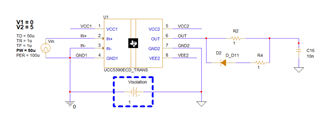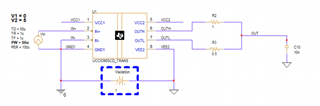Using PSpice for TI, simulations were run to measure the voltage, current and power dissipation for the S and E version with the following specifications:

• Supply Voltage: +15 V, 0 V
• Gate Resistor: (1 ohm, 2.5 ohms, 5 ohms, and 10 ohms) Rgoff is ½ of the Rgon resistance
• Frequency: 10 kHz

The choice of the external gate drive resistor plays a crucial role in the gate driver behavior, such as to limit noise and ringing. Additionally, a gate resistor is important to limit the peak current from the gate driver in order to control the slew rate of the IGBT or MOSFET that it drives. The selection of the external gate drive resistor will affect three things: drive current, gate-drive power dissipation, and rise and fall times. The key difference between the versions E and S is how the gate resistors for turn-on and turn-off are connected from the output to the gate of the MOSFET or IGBT.

How is gate current impacted by gate resistance?
Using PSpice for TI, the drive current for E vs. S versions were measured across varying gate resistances, as shown in the figure below. To see if there are any significant difference between the E and S version.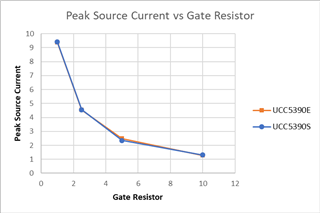Figure 1: Peak Source Current vs Gate Resistor

From figure 1, there are no significant differences in the peak source current between the S and E versions. The gate current is most impacted by the external gate resistor, where larger resistance results in lower peak gate current. The equation below found in section 11.2.2.2 in the data sheet explains why the peak source current decreases when the gate resistor increases. As the external turn-on resistance RON and the power transistor internal gate resistance RGFET(INT) reduces the peak source current as shown in the equation below.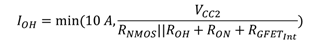• RONis the external turn-on resistance.
• RGFET_Intis the power transistor internal gate resistance, found in the power transistor data sheet.
• IOHis the peak source current which is the minimum value between 10 A, the gate-driver peak source current, and the calculated value based on the gate-drive loop resistance.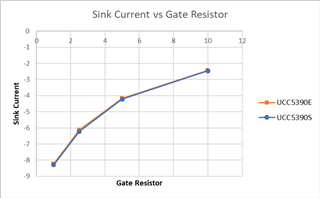Figure 2:Sink Current vs Gate Resistor

Similarly, figure 2 shows that there are no differences in the sink source current between the S & E version. As the gate resistor increases, the absolute sink source current decreases. The equation below is used to calculate the sink source current. The external turn-off resistance ROFF and the power transistor internal gate resistance RGFET(INT) reduces the peak sink current as shown in the equation below.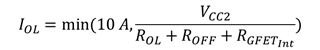• ROFFis the external turnoff resistance.
• IOLis the peak sink current which is the minimum value between 10 A, the gate-driver peak sink current, and the calculated value based on the gate-drive loop resistance.

How is the gate resistor peak power dissipation impacted by varying gate resistance?

The power dissipated is the product of the current and voltage. As we increase the value of the gate resistor, the value of the current decreases. Since, the power dissipated is the product of the current and voltage, hence the power dissipation decreases as the current decreases. The figure below shows the peak power dissipation across varying gate resistor to see the differences between the S & E version.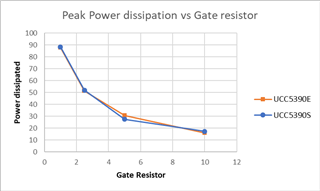Figure 3: Peak power dissipation across varying gate resistors

From figure 3, there are no significant differences in the peak power dissipation between the S and E variants. The power dissipation is most impacted by the external gate resistor, where larger resistance results in lower power dissipation.

It’s important to note that duration of the peak power dissipation increases as the gate resistor increases as shown in figure 4. In addition, there are no significant differences in the duration of the peak power dissipation between the E & S versions.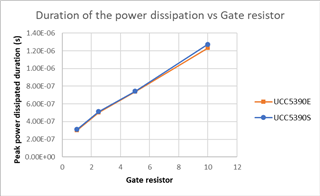Figure 4: Duration of the power dissipation vs varying gate resistors

Does the external diode for the E version affects the total power dissipation?

The UCC53x0E has one pin which requires the customer the need of an external diode at the output to achieve separate turn-on and turn-off paths. Table 1 shows the average current, the voltage difference between (VCC2, VEE2), and the average power dissipated through the IC chip between the S & E version.

 UCC5390E UCC5390S Average Current 0.06 A 0.06 A Voltage 14.82 V 14.89 V Average Power dissipated 0.87 W 0.87 W

Table 1: Total average power dissipation of the IC chip

Diode specifications:

As mentioned earlier, the E version requires the customer to add an external diode at the output to have a separate turn-on and turn-off paths. Since, the diode is needed in the turn-off region, the external turnoff resistance (ROFF) impacts the current passing through the resistor. Table 2 shows the measured reverse voltage, forward voltage, and non-repetitive peak sure forward current across varying gate resistors.

 Gate resistor (Ω) Forward Voltage (V) Non-repetitive peak surge forward current (A) 1 0.261 3.99 2.5 0.259 3.01 5 0.256 2.05 10 0.252 1.21

Table 2: Gate resistor effect on diode

Therefore, the customer should pay attention for diode specifications, rating, and parameters with respect to the gate resistor when choosing a diode. Additionally, the forward voltage should be minimized when choosing a diode. The forward voltage drop corresponds to the decrease of the effective turn-off voltage (gate to source). Table 3, shows diode ratings for gate resistor ranging from 1 ohm to 10 ohms.

 Parameter Ratings Reverse Voltage > (VCC2-VEE2) Non-repetitive peak surge forward current > 3.99 A

Table 3: Diode Rating

XBS104S13R-G and MURS160T3G are diode that meet the criteria from table 3 for the customer. XBS104S13R-G costs \$0.17 per 1ku while MURS160T3G costs \$0.15 per 1ku.

Key points:

• No significant differences between the S & E version in (Gate Voltage, Gate Current, and Power Dissipation).
• As the value of the External Gate drive resistor increases the value of gate current decreases.
• As the value of the External Gate drive resistor increases, the peak power dissipation decreases, yet the duration of the peak power dissipation increases.
• Customization:
• Split output has two pins which allows for separate turn-on/off paths and control over the driver source/sink current.
• Single output has one pin which needs an external diode at the output to achieve separate turn-on/off paths.
• Cost:
• Added Diode for the E version which accounts to 17.3%| (1 ku) additional cost of a gate driver.
• Board Area
• The added diode would take more space in the circuit

Conclusion:

A single output gate driver has one pin (VOUT) which requires the customer to add a diode at the output for turn-on and turn-off. This would increase the bill of material cost, board area, and adds impedance in the gate loop. The forward voltage drop of the diode corresponds to the decrease of the effective turn-off voltage (gate to source).

While a split output has two pins which leads to separate turn-on and turn-off path and control over the drive source/sink current. This saves the use of the external diode at the output which decrease the bill of material for the customer compared to the single output gate driver.